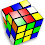## Thursday, January 17, 2008

### How about a math puzzle?

It has been awhile since I've posted any non-NPR puzzles. Here's one to make you think:
Q: A nine-digit number ABCDEFGHI is such that its digits are all distinct and non-zero. It has the following properties:
The two-digit number AB is divisible by 2,
the three-digit number ABC is divisible by 3,
the four-digit number ABCD is divisible by 4,
and so on until finally,
the nine-digit number ABCDEFGHI is divisible by 9.

What is this special nine-digit number?

1.I couldn't figure out a shortcut to determining the number, so I ended up making a computer program to do the work for me.

Though crudely written, it did find the answer for me.

The number is 381654729

2.2 x 19 = 38
3 x 127 = 381
4 x 954 = 3816
5 x 7633 = 38165
6 x 63609 = 381654
7 x 545221 = 3816547
8 x 4770684 = 38165472
9 x 42406081 = 381654729

However, you can get there with logic rather than a brute force program:

We know that ABCDE must be a multiple of 5, so E must be 5.

AB, ABCD, ABCDEF, ABCDEFGH are multiples of 2, 4, 6 and 8 respectively. Thus their last digits (B, D, F and H) must be even. That leaves 1, 3, 7 and 9 for A, C, G and I.

ABCD is a multiple of 4, so CD must be a multiple of 4. Given that C is odd, the possible values are {12, 16, 32, 36, 72, 76, 92 and 96}. That means D must be 2 or 6.

Similarly ABCDEFGH is a multiple of 8 (and therefore 4). Using similar logic, GH will be in {12, 16, 32, 36, 72, 76, 92 and 96}. Therefore H must be 2 or 6.

Because 2 and 6 are accounted for, B and F must be 4 and 8. That means FGH will be {412, 416, 432, 436, 472, 476, 492, 496, 812, 816, 832, 836, 872, 876, 892 or 896}.

But FGH needs to be a multiple of 8 as well as a multiple of 4. The only multiples of 8 are {416, 472, 496, 816, 872 or 896}

ABC is a multiple of 3 so its digits must add to be a multiple of 3. Possible choices are {147, 183, 189, 381, 387, 741, 783, 789, 981, 987}

ABCDEF is a multiple of 6 and therefore also a multiple of 3. Given that ABC already adds to a multiple of 3, we only need to check DEF. E is 5. Possible choices for DEF are {258, 654}

Putting things together we have a couple possible patterns:
A-4-C-2-5-8-G-6-I
or
A-8-C-6-5-4-G-2-I

That reduces the set of choices for FGH to {472, 816, 896}

So the 3 possible patterns are now:
A-4-C-2-5-8-1-6-I
A-4-C-2-5-8-9-6-I
A-8-C-6-5-4-7-2-I

Looking at our set of possible values for ABC we have {147, 183, 189, 381, 387, 741, 783, 789, 981, 987}. If B = 4, then ABC is either {147 or 741}. That eliminates the first string. If B = 8, all the values using 7 are eliminated.

So now we have:

{147 or 741}
1-4-7-2-5-8-9-6-I
7-4-1-2-5-8-9-6-I

{183, 189, 381, 981}
1-8-3-6-5-4-7-2-I
1-8-9-6-5-4-7-2-I
3-8-1-6-5-4-7-2-I
9-8-1-6-5-4-7-2-I

Okay there are 6 sequences to check to see if ABCDEFG is a multiple of 7.
1472589 / 7 = 210369.857142...
7412589 / 7 = 1058941.285714...
1836547 / 7 = 262363.857142...
1896547 / 7 = 270935.285714...
3816547 / 7 = 545221
9816547 / 7 = 1402363.857142...

As you can see only one is evenly divisable by 7.
3816547

That's part of this pattern:
3-8-1-6-5-4-7-2-I

The final digit is 9. Obviously the nine digits add up to 45, so the full number will be a multiple of nine regardless.